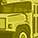Level 2
Level 2# Tiny distance calculation

Hi,

Now, I try to calculate the change of tiny distances with the phase differences of chirps. I have got the figure of phase differences.  Can I directly calculate the distances with the formula:  Delta phase = 4*pi*Delta distance/lambda, where lambda is the wavelength. You can see the figure.1 below. Then, I configure the radar as figure.2, how can I calculate the start frequency? I do not know the default center_frequency_HZ. Hope your patient answers. I will appreciate a lot if you can answer this technical details.

1 SolutionModeratorModerator# Re: Tiny distance calculation

Hello @shibiana ,

The FMCW system measures the instantaneous difference between the transmitted and reflected signal frequencies δf, which is directly proportional to the time difference δt of the reflected chirp. The time difference can be used to calculate the range of the target. If there are several targets with the same distance, we cannot distinguish them by the range-frequency relationship because they have the same IF frequency in the Spectrum. Thus, we can detect these small scale movements and distinguish different targets by measuring the phase change of IF signals. Please refer to the following links for the basic idea of how it is done, it also has the python source code for reference

You may also refer to the documentation for detailed explanation on the radar parameters from \radar_sdk\radar_sdk\doc. Regarding the center frequency, center frequency is calculated as = (Higher frequency - Lower frequency)/2 . Then you add the answer to the lower frequency to get the center frequency value.

Hope this helps!

Best Regards,
Honey

3 RepliesModeratorModerator# Re: Tiny distance calculation

Hello @shibiana ,

The FMCW system measures the instantaneous difference between the transmitted and reflected signal frequencies δf, which is directly proportional to the time difference δt of the reflected chirp. The time difference can be used to calculate the range of the target. If there are several targets with the same distance, we cannot distinguish them by the range-frequency relationship because they have the same IF frequency in the Spectrum. Thus, we can detect these small scale movements and distinguish different targets by measuring the phase change of IF signals. Please refer to the following links for the basic idea of how it is done, it also has the python source code for reference

You may also refer to the documentation for detailed explanation on the radar parameters from \radar_sdk\radar_sdk\doc. Regarding the center frequency, center frequency is calculated as = (Higher frequency - Lower frequency)/2 . Then you add the answer to the lower frequency to get the center frequency value.

Hope this helps!

Best Regards,
HoneyLevel 2
Level 2# Re: Tiny distance calculation

Sorry, I have already known the center frequency. However, about the tiny distance calculation I still have some problems. Actually, I want to calculate the tiny displacement of breathing. Now, I have already got the time-phase figure of breathing (Fig.1), and I can also derive the phase difference figure with Fig.1. But I do not know how to transfer the phase information into displacement.ModeratorModerator# Re: Tiny distance calculation